# OTDR: Two-Way Optical Fiber Measurement

When light is injected into an optical fiber, some of it is scattered within the optical fiber to the opposite end of the light‘s path. This is called backscattered light. Each optical fiber has its own backscattered light value, or level.　Since the connection loss for fibers with different backscatter levels varies according to the measurement direction, correct loss assessment requires measurement from both ends.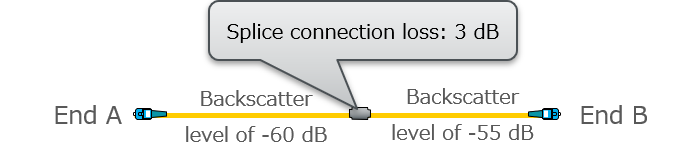For example, a fiber whose backscatter level is -60 dB is connected to a fiber of -55 dB at a loss of 3 dB: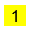When an Optical Time Domain Reflectometer (OTDR) measures from End A, the light travels at a backscatter level of  -60 dB and then declines by 3 dB at the connection point. However, since the backscatter level beyond the connection point is -55 dB, which is higher than the A side by 5 dB, the backscatter level is displayed as 2 dB due to the difference between the connection loss and the backscatter level of the B side. The loss of event 1 is -2 dB, which looks like the light is amplified. This type of event is also referred to as a “gainer”.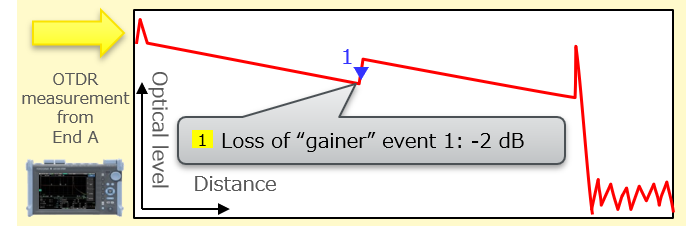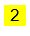On the other hand, when the OTDR measures from End B, the light travels at a backscatter level of -55 dB and declines by 3 dB at the connection point. However, since the backscatter level beyond the connection point is -60 dB, the light declines by an additional 5 dB. It therefore declines 8 dB in total. The loss of event 1 is displayed, in fact, as 8 dB. Conversely this type of event is also referred to be a “loser”

The correct loss value is the average of the following:

The loss of “gainer” event 1 measured from End A: -2 dB

The loss of “loser” event 1 measured from End B: 8 dB

Therefore, the correct loss =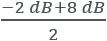= 3 dB

By averaging the values measured from both ends, the backscatter value of the fibers is deducted and the loss of event 1 is corrected. Analysis of two-way measurement in the AQ7280 and AQ1210 is [Advanced Analysis] [2-Way Trace].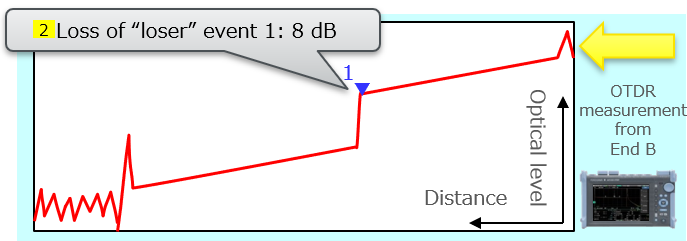### Related Products & Solutions

#### AQ1210 Series Optical Time Domain Reflectometer

• Yokogawa AQ1210 series OTDR
• Fast and precise
• Optical time domain reflectometer delivers high reliability
• Harsh field conditions
• Multi-touch touchscreen, automatic measurement

#### AQ7280 Modular Optical Time Domain Reflectometer

• Yokogawa AQ7280 Optical Time Domain Reflectometer (OTDR)
• Fast and precise measurements with confidence
• Satisfies broad range of test and measurement needs